# Magnetic moment in terms of magnetization

• 15
•
• 1
•
•
•
•
•
16
Shares

## Magnetic moment and magnetization

### Magnetic moment

Do we know that what is the magnetic moment? Let’s take a small snapshot of the magnetic moment. Actually, the magnetic moment also known as the magnetic dipole moment is the magnetic strength and orientation of a magnet or any other object that produces a magnetic field. In simple words, it is the ability of a magnetic material to align itself in such a way that it can experience zero torque.

Some students are confused with the magnetic moment and magnetic dipole moment, you don’t need to confuse these terms because they are the same thing. The magnetic moment is just the movement of magnetic materials in a magnetic field. If a magnetic dipole show movement in the magnetic field then we say magnetic dipole moment. Magnetic dipole moment is nothing but the product of the pole strength of either side (north pole or south pole) and the separation distance between them. Mathematically, it is given as-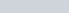where μ is the magnetic moment andis the magnetic pole strength.

Here, in this present article, we will discuss the relationship between the magnetic moment and magnetization. So let’s get started…

### Relation with magnetization

As we know that the magnetic moment is a quantity that describes the ability of the magnetic material to show orientation in the uniform magnetic field. But, can you tell me that does all the portion of magnetic materials produces the same quantity of magnetic moment. Obviously not! Because each portion of the magnet would have a different volume.

Sometimes, it is useful or become necessary to know that how much of the net magnetic moment of the object is produced by a particular portion of the magnet. Therefore, it is useful to define the term magnetization field M as-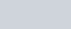where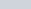is the magnetic dipole moment and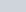is the volume of a infinitesimal small portion of the magnet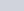. In the form of derivative this equation is represented as-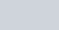where dm is the elementary magnetic moment and dV is the volume element. The net magnetic moment of the magnet m can be given as-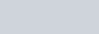where the triple integral denotes integration over the volume of the magnet.

For uniform magnetization (where both the magnitude and the direction of the M is the same for the whole magnet (for example: a straight bar magnet) the above equation can be written as: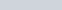where V is the volume of the bar magnet. For commercially available ferromagnetic materials, magnetization is not listed as material parameter. It is listed as residual flux density (or remanence), denoted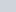. The formula for magnetic moment needed in this case can be calculated as-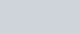where

•is the residual flux density, in tesla.
• V is the volume of the magnet in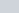.
•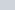is the permeability of the free space, whose value is (4π×10−7 H/m)

Stay tuned with Laws Of Nature for more useful and interesting content.